# Dynamic Sheet Reference with INDIRECT – Excel & Google Sheets

This tutorial will demonstrate how to use the INDIRECT Function to reference sheets dynamically.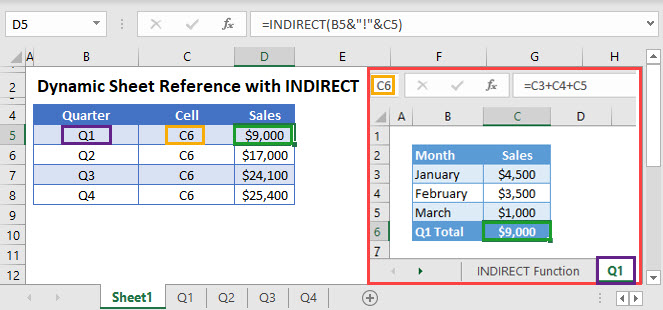## Dynamic Sheet Reference using the INDIRECT Function

The INDIRECT Function allows you to reference a range with a string of text, making it very easy to change the cell reference. Its syntax is: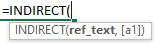This example will reference cell C6 in worksheets Q1, Q2, Q3, and Q4:

``=INDIRECT(B3&"!"&C3)``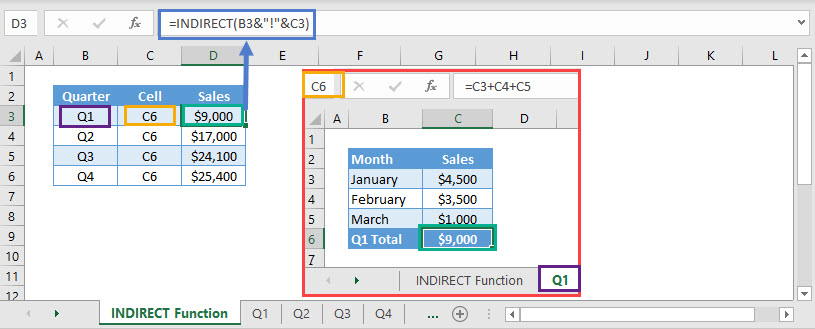In the above example, the Concatenate Operator (&) has been nested in the INDIRECT function to join the worksheet name and cell location.

### INDIRECT – Volatile Function

Warning: The INDIRECT Function is volatile. This means it re-calculates every time the workbook re-calculates. If you rely on too many INDIRECT functions, it will negatively impact your workbook speed.

Usually, you can use other non-volatile functions instead of INDIRECT. One such function is the CHOOSE Function.

## CHOOSE Function – Sheet Reference

The CHOOSE Function returns a value from a range or list of values based on a given position. Its syntax is: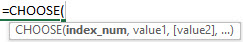For eg. CHOOSE(2,”Joseph”,”Rachel”,”John”) would return “Rachel”, as it’s the 2nd value listed after the index number.

The following example will reference total sales’ values for each quarter from worksheets Q1, Q2, Q3 and Q4.

``=CHOOSE(C3,'Q1'!\$C\$6,'Q2'!\$C\$6,'Q3'!\$C\$6,'Q4'!\$C\$6)``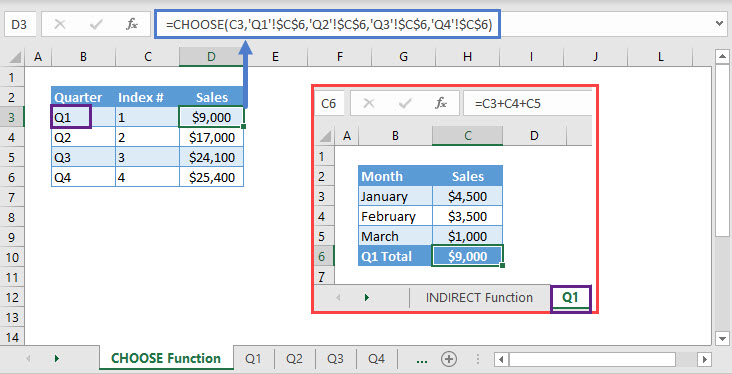In this example, column C specifies the position of the required value. Internally, the formula allots positions in the same order as selected, in other words, it’s the values’ position from the index number.

Note: If the number specified for the ‘index_num‘ argument is greater than the total number of values, Excel would return #VALUE! Error.

## Dynamic Sheet Reference with INDIRECT in Google Sheets

These formulas work exactly the same in Google Sheets as in Excel.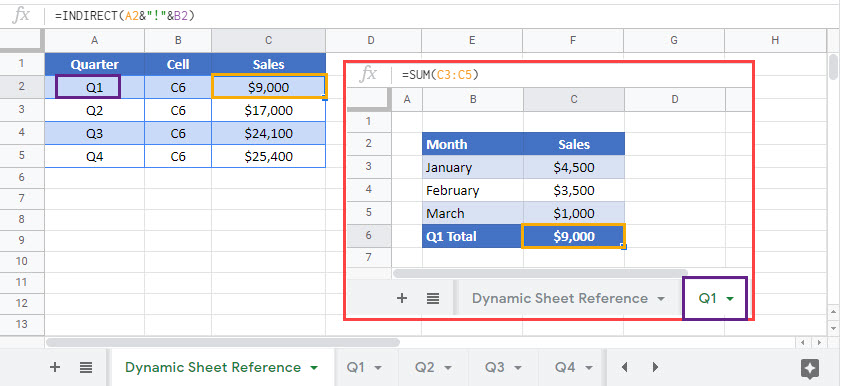### Excel Practice Worksheet

Practice Excel functions and formulas with our 100% free practice worksheets!